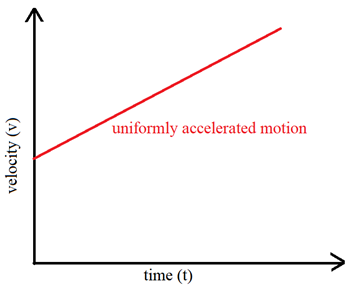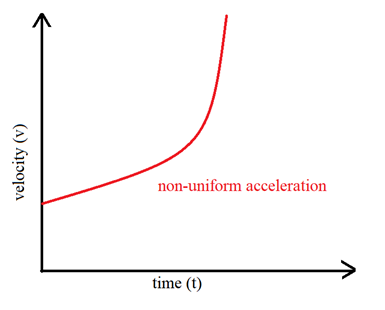# Rate of Change of Velocity

The rate of change of velocity is called acceleration. A body moving at any speed can increase or decrease its speed. This process is called acceleration, if its speed increases and deceleration, if its speed decreases. Velocity-time graphs are a good method to analyse this phenomenon. In velocity-time graphs, the slope of graph gives the acceleration of the body.  It shows how the velocity of a body is changing with time.

Suppose a car starts off from rest (i.e. initial velocity is zero) and its velocity increases at a steady rate so that after 5 seconds its velocity is 10 m/s . Now, in 5 seconds the velocity has increased by 10 – 0 = 10 metres per second and in 1 second the velocity increases by 10/5 = 2 metres per second. In other words, the rate at which the velocity increases is 2 metres per second every second. The car is said to have an acceleration of 2 metres per second per second.

This gives us the definition of acceleration:

Acceleration of a body is defined as the rate of change of its velocity with time.

Acceleration = (Change in Velocity)/(Time taken for Change)

Now, the change in velocity is the difference between the final velocity and the initial velocity. That is,

change in velocity = final velocity – initial velocity

Acceleration = (Final velocity – Initial velocity)/(Time taken)

So, let us suppose that the initial velocity of a body is  and it changes to a final velocity ‘v’ in time ‘t’, then:

a = (v-u)/t

Where, a = acceleration of the body

v = final velocity of the body

u = initial velocity of the body

t = time taken for the change in velocity

Since, acceleration is the change in velocity divided by time, therefore, the unit of acceleration will also be the unit of velocity (metres per second) divided by the unit of time (second). Thus, the SI unit of acceleration is “metres per second” which is written as m/s2 or ms-2. The other units of acceleration which are also sometimes used are “centimetres per second square” (cm/s2 or cm s-2) and “kilometres per hour square” (km/h2 or km h-2).

If the motion is in a straight line, acceleration takes place in the direction of velocity, therefore, acceleration is a vector quantity. It is to be understood from the definition of acceleration that since, acceleration = (v-u)/t , a body moving with uniform velocity has zero acceleration because the change in velocity, (v-u) = 0 for a body moving with uniform velocity.

From the above discussion we can conclude that –

• When the body is moving with uniform velocity, acceleration is zero.
• When the velocity of a body is not uniform (it is changing), the motion is accelerated.

Now, we will discuss the types of acceleration. A body can increase/decrease its velocity by either equal amounts in equal intervals of time or by unequal intervals in equal intervals of time. So, on the basis of this, there are three types of acceleration – uniform acceleration, non-uniform acceleration and retardation (or negative acceleration or deceleration).

### Uniform acceleration or Uniform Rate of Change of Velocity

When the velocity of a car increases, the car is said to be accelerating. If the velocity increases at a uniform rate, the acceleration is said to be uniform. Hence, we arrived at a definition for uniform acceleration:

A body has a uniform acceleration if it travels in a straight line and its velocity increases by equal amounts in equal intervals of time.

In other words, a body has a uniform acceleration if its velocity changes at a uniform rate.

We can easily understand this type of acceleration through some example :-

• The motion of a freely falling body is an example of uniformly accelerated motion.
• The motion of a bicycle going down the slope of a road when the rider is not pedalling and wind resistance is negligible, is also an example of uniformly accelerated motion.
• The motion of a ball rolling down an inclined plane is an example of uniformly accelerated motion.

The velocity-time graph of a uniformly accelerated body is a straight line.Note that, the three equations of motion are defined only for uniformly accelerated motion.

### Non-uniform acceleration or Non-uniform Rate of Change of Velocity

A body has a non-uniform acceleration if its velocity increases by unequal amounts in equal intervals of time. In other words, a body has a non-uniform acceleration if its velocity changes at a non-uniform rate.

The speed (or velocity) of a car running on a crowded city road changes continuously. A car maybe moving at constant speed for some time and then can increase its speed for some time or can decrease its speed for some other moment of time. hence, the velocity-time graph of a body moving with non-uniform acceleration is a curved line.### Retardation or Deceleration

Acceleration takes place when the velocity of a body changes. The velocity of a body may also decrease hence, another type of acceleration is negative acceleration or retardation. If the velocity of a body increases, the acceleration is positive and if the velocity of a body decreases the acceleration is negative. A body is said to be retarded if its velocity is decreasing.

Retardation is measured in the same way as acceleration because retardation is actually acceleration with negative sign.

Retardation = (Change in Velocity)/(Time taken)

Acceleration, a = (Final Velocity – Initial Velocity)/(Time taken)

Example – a train is retarded when it slows down on approaching a station because then its velocity decreases.

Conclusion – The rate of change of velocity is called acceleration. it is of three types – uniform acceleration, non-uniform acceleration and retardation. If the velocity of a body increases by equal amounts in equal time intervals then it is called uniform acceleration. if the velocity of a body increases by unequal amounts in equal time intervals then it is called non-uniform acceleration. If the speed of a body decreases in given time then it is called retardation. The velocity-time graph of uniform acceleration is a straight line. The velocity -time graph of non-uniform acceleration is curved line.

Click here for NCERT Solutions for Class 9